# Fluids

Student Learning Objectives
Lessons / Lecture Notes
Important Equations
Example Problems
Applets and Animations

Student Learning Objectives

• To understand the concept of mass density.
• To understand pressure in liquids and gases.
• To use Archimedes’ principle to understand buoyancy.
• To use an ideal-fluid model to study fluid flow.
• To understand the role of viscosity in the flow of realistic fluids.

Lessons / Lecture Notes

PY105 Notes from Boston University (algebra-based):

Introductory physics notes from University of Winnipeg (algebra-based):

Physics 2A notes from Dr. Bobby W.S. Lau (algebra-based)

HyperPhysics (calculus-based)

Important Equations (for algebra-based Physics)

Example Problems

Example Problems for algebra-based physics (from College Physics 2nd Edition by Knight, Jones, and Field):

Example Problems (Fluids)
Solutions to Example Problems (Fluids)

Applets and Animations

 Density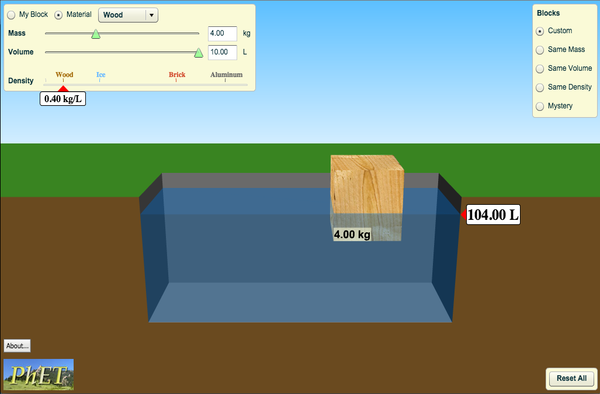Why do objects like wood float in water? Does it depend on size? Create a custom object to explore the effects of mass and volume on density. Can you discover the relationship? Use the scale to measure the mass of an object, then hold the object under water to measure its volume. Can you identify all the mystery objects? Balloons & Bouyancy / Gas Pressure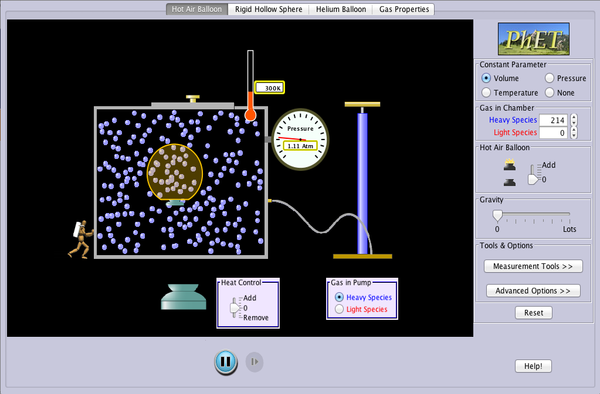Experiment with a helium balloon, a hot air balloon, or a rigid sphere filled with different gases. Discover what makes some balloons float and others sink. Check out the Gas Properties tab to see a nice example of the different factors that affect gas pressure. Gas Pressure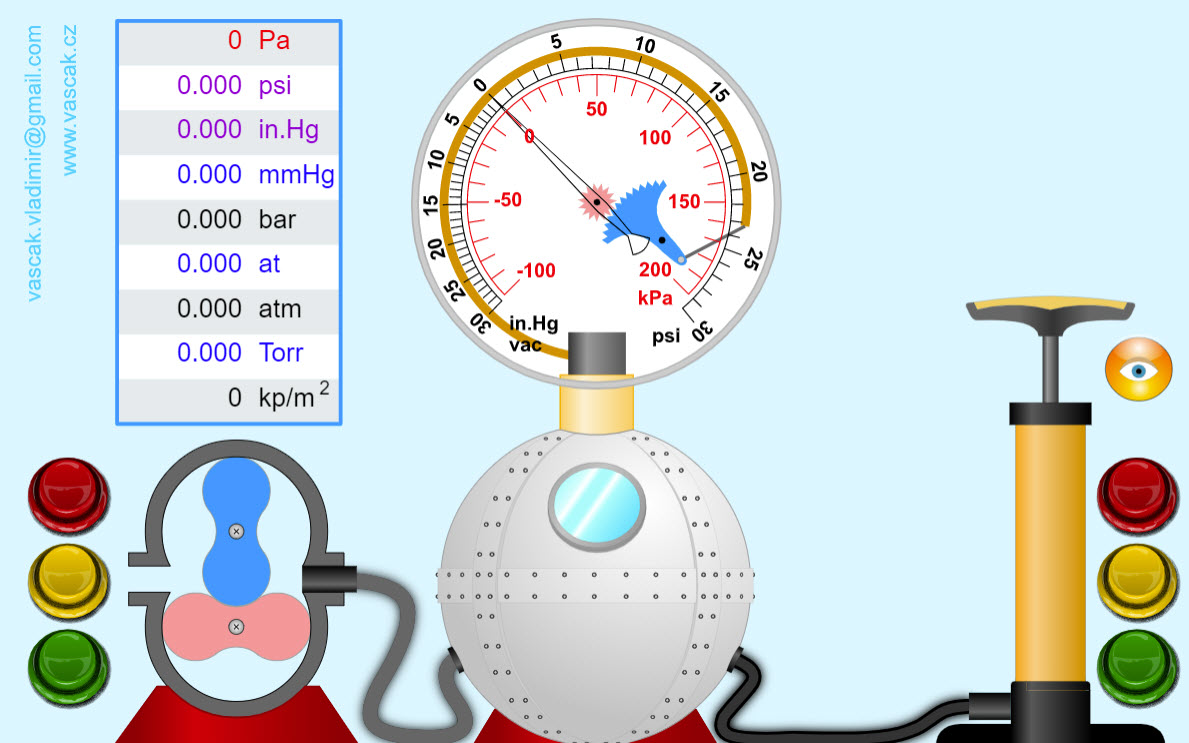A nice animation showing how an air pump works. Under Pressure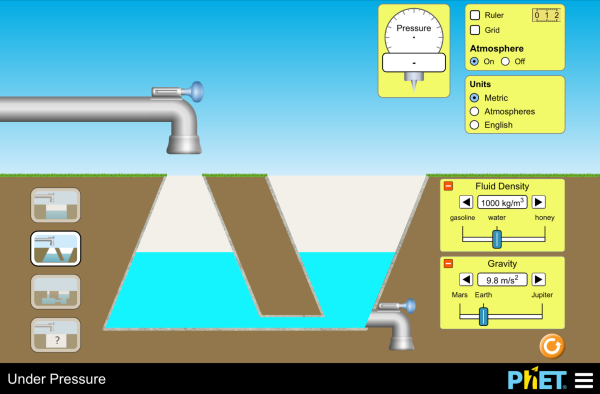Explore pressure under and above water. See how pressure changes as you change fluids, gravity, container shapes, and volume. Pressure in a Static Fluid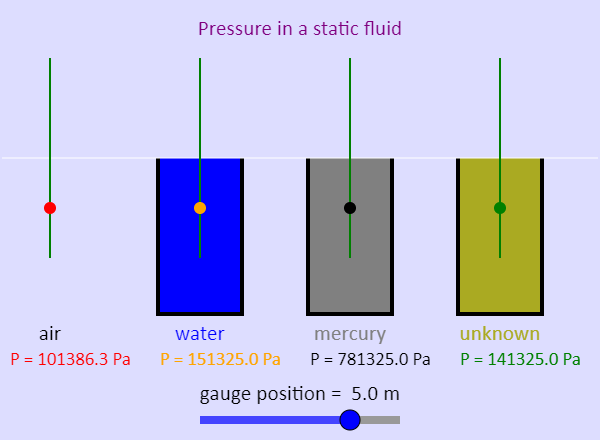This simulation shows four pressure sensors. One stays in air, the second can go into a container of water, the third can go into a container of mercury, and the fourth can go into a container of unknown fluid. The slider controls the vertical position of the pressure gauges. The horizontal white line shows the position of standard atmospheric pressure, which is at the top of each fluid. Note what happens to the pressure readings as you move the pressure gauges up and down. You can choose between displaying absolute pressure or gauge pressure - gauge pressure is simply the absolute pressure minus atmospheric pressure (101325 Pa). With this simulation, what can you determine about the unknown fluid? Bouyancy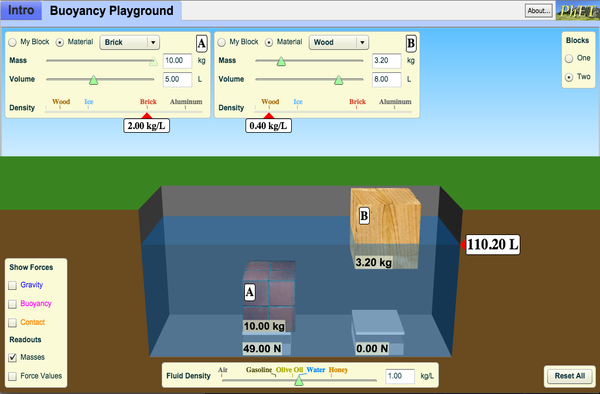When will objects float and when will they sink? Learn how buoyancy works with blocks. Arrows show the applied forces, and you can modify the properties of the blocks and the fluid. Archimmede's Principle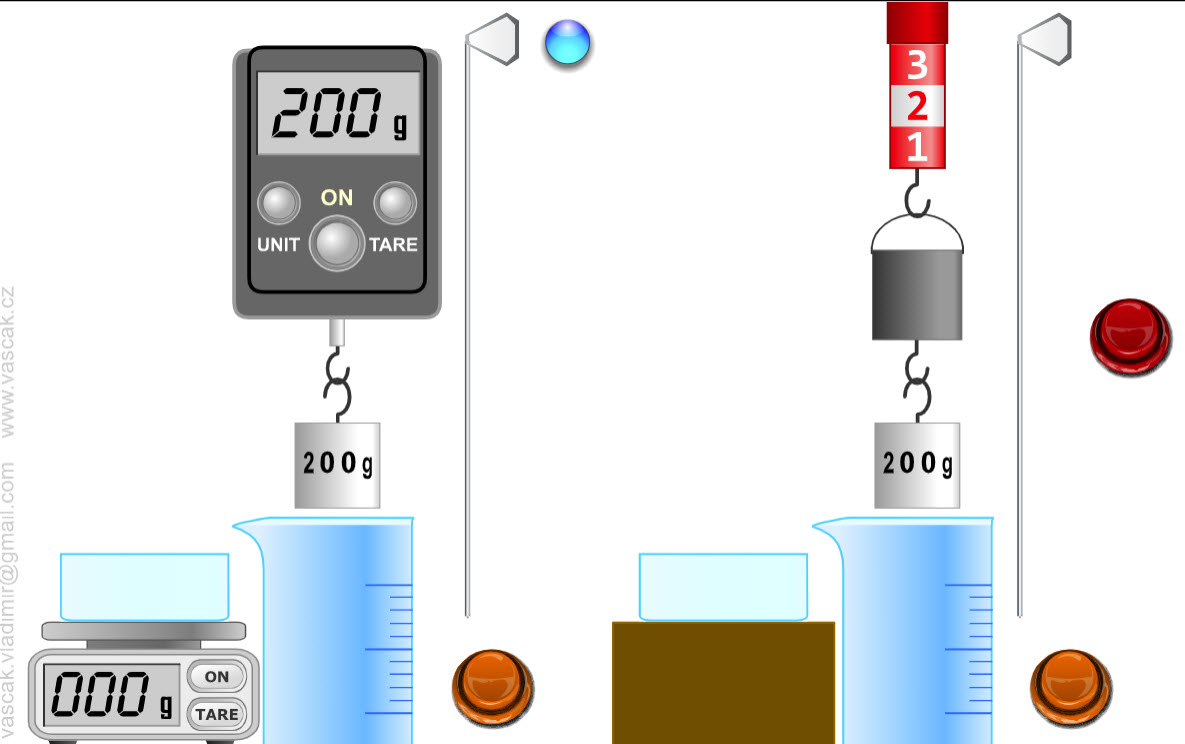A nice animation demonstrating Archimede's Principle. Bouyant Force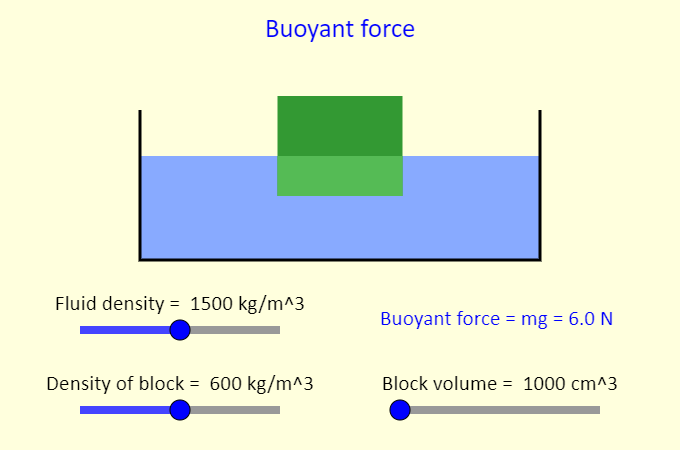This simulation illustrates the concept of the buoyant force. The buoyant force is the upward force exerted on an object by a fluid when the object is partly or entirely immersed in the fluid. In this case we place a low-density block in a container of fluid. The block floats with some fraction submerged (the fraction submerged is the block density divided by the fluid density). The buoyant force equals the weight of the block, because the block is in equilibrium. However, the buoyant force is also given by Archimedes' principle, which is that the buoyant force is equal to the weight of the fluid displaced (which itself is equal to the density of the fluid multiplied by g multiplied by the volume of fluid displaced by the object). Investigate what the buoyant force depends on (and what it does not depend on). Note that the simulation sets g to be 10 N/kg. Bouyant Force in Liquids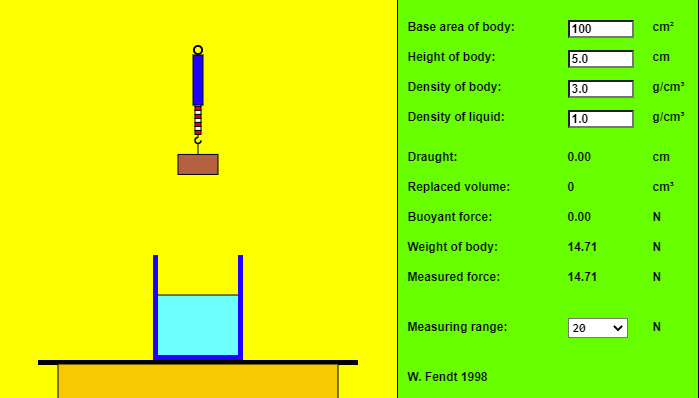This HTML5 app shows a simple experiment concerning the buoyancy in a liquid: A solid body hanging from a spring balance is dipped into a liquid (by dragging the mouse!). In this case the measured force, which is equal to the difference of weight and buoyant force, is reduced. You can change (within certain limits) the preselected values of base area, height and densities by using the appropriate text fields. After you have pressed the "Enter" key, the program will indicate the new values of depth, replaced volume, buoyant force, weight and measured force. A gravitational acceleration of g = 9.81 m/s2 was presupposed. If you see the words "Maximum exceeded!" (red letters), you have to choose an adequate measuring range. Apparent Weight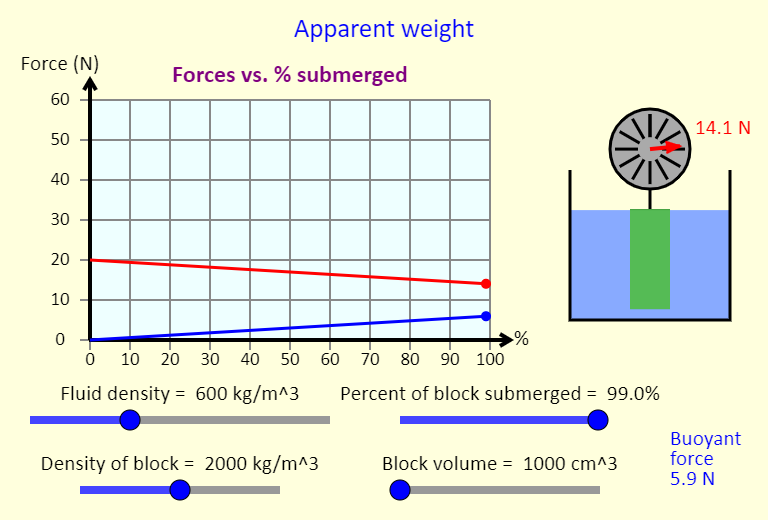This simulation illustrates the concept of apparent weight, as well as the buoyant force. The buoyant force is the upward force exerted on an object by a fluid when the object is partly or entirely immersed in the fluid. In this case there is a block hanging from a spring scale. The block can be lowered into the fluid (use the "Percent of block submerged" slider). This results in the spring scale reading changing - why? The spring scale reading shows the block's apparent weight - this is onlt equal to the actual weight of the block when the block is completely out of the fluid. Investigate what the buoyant force depends on (and what it does not depend on). Note that the simulation sets g to be 10 N/kg. Given the limits on the sliders in the simulation, what is the biggest buoyant force that can be achieved here? You can also show a scale under the container of fluid. It is interesting to see what happens to the scale reading as the block is lowered into (or removed from) the fluid. Cartesian Diver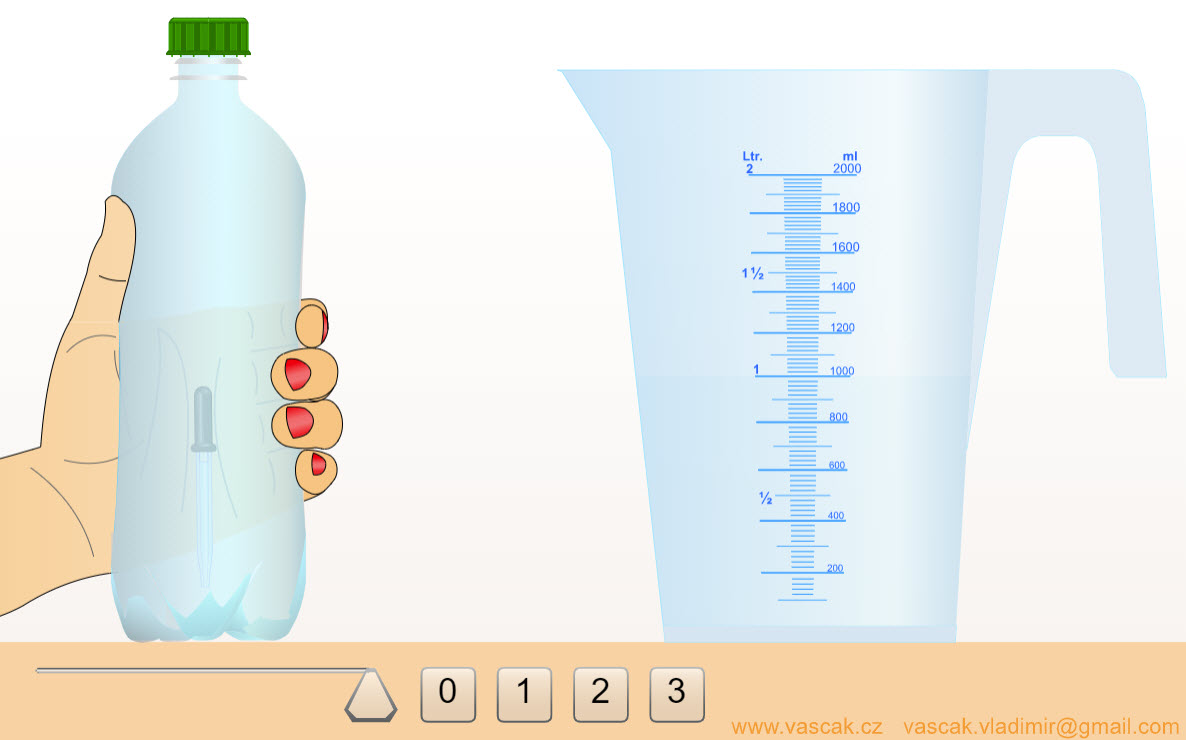This simple animation demonstrates how a Cartesian diver works. Fluid in a Pipe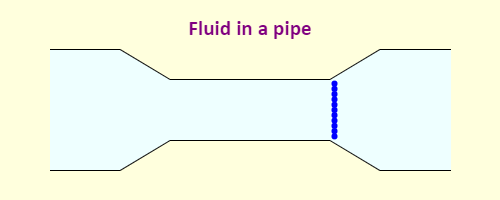Simple animation showing how a fluid flows in a pipe when the area of the pipe changes. Fluid Pressure and Flow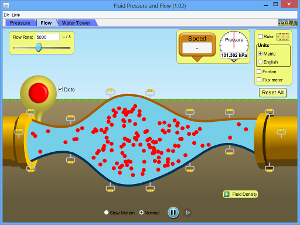Explore pressure in the atmosphere and underwater. Reshape a pipe to see how it changes fluid flow speed. Experiment with a leaky water tower to see how the height and water level determine the water trajectory. Bernoulli's Principle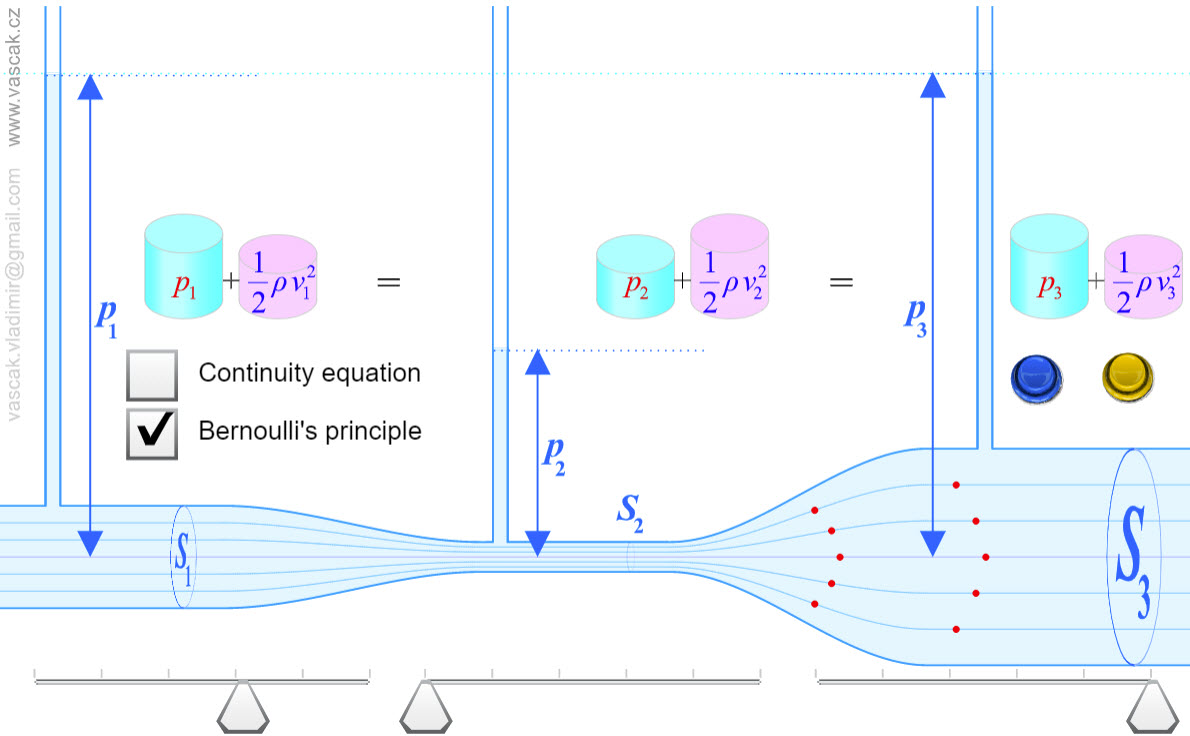A very nice applet demonstrating Bernoulli's Principle. Wings and Lift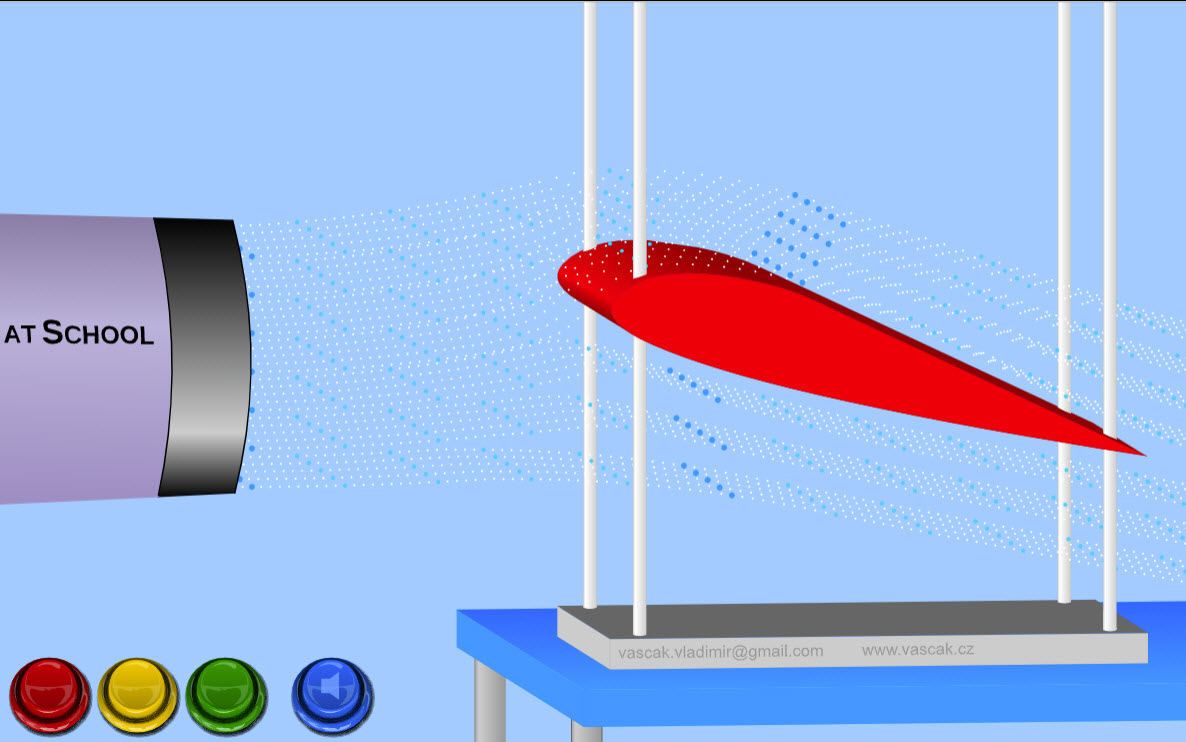This cool animation shows how a wing generates lift by deflecting the air molecules as they travel over the wing. There's a Hole in My Bucket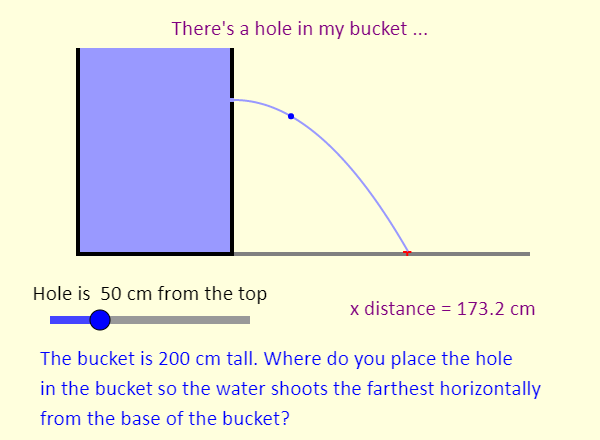This simulation shows a bucket full of water. The bucket has a hole in its right side - you can use the slider to change the location of the hole. Where do you think the hole should be placed so that the water shoots out farthest horizontally from the base of the bucket? This problem actually combines fluids with ideas from projectile motion. You can use Bernoulli's equation to determine how fast the fluid emerges from the hole - we assume the fluid has no viscosity and that the velocity of the fluid is entirely horizontal when it emerges from the hole. Then, you can determine how long it takes to fall to the level of the bottom of the bucket, and how far it travels horizontally in that time. So, it's a nice combination of concepts. Notice how the maximum horizontal distance compares to the height of the bucket, and also see if you can spot a symmetry here in how far horizontally the water travels as a function of the position of the hole. DiffusionMix two gases to explore diffusion! Experiment with concentration, temperature, mass, and radius and determine how these factors affect the rate of diffusion.